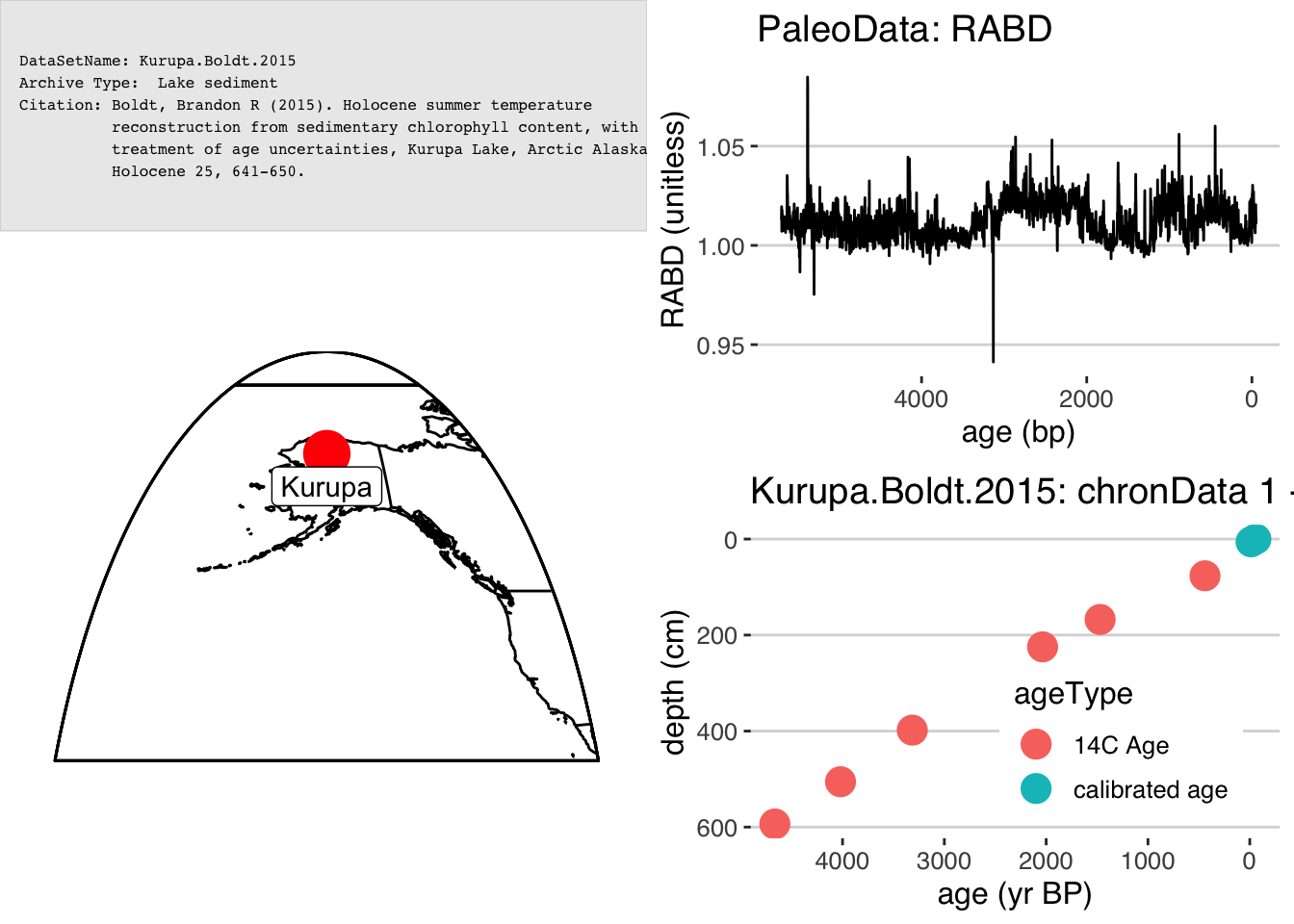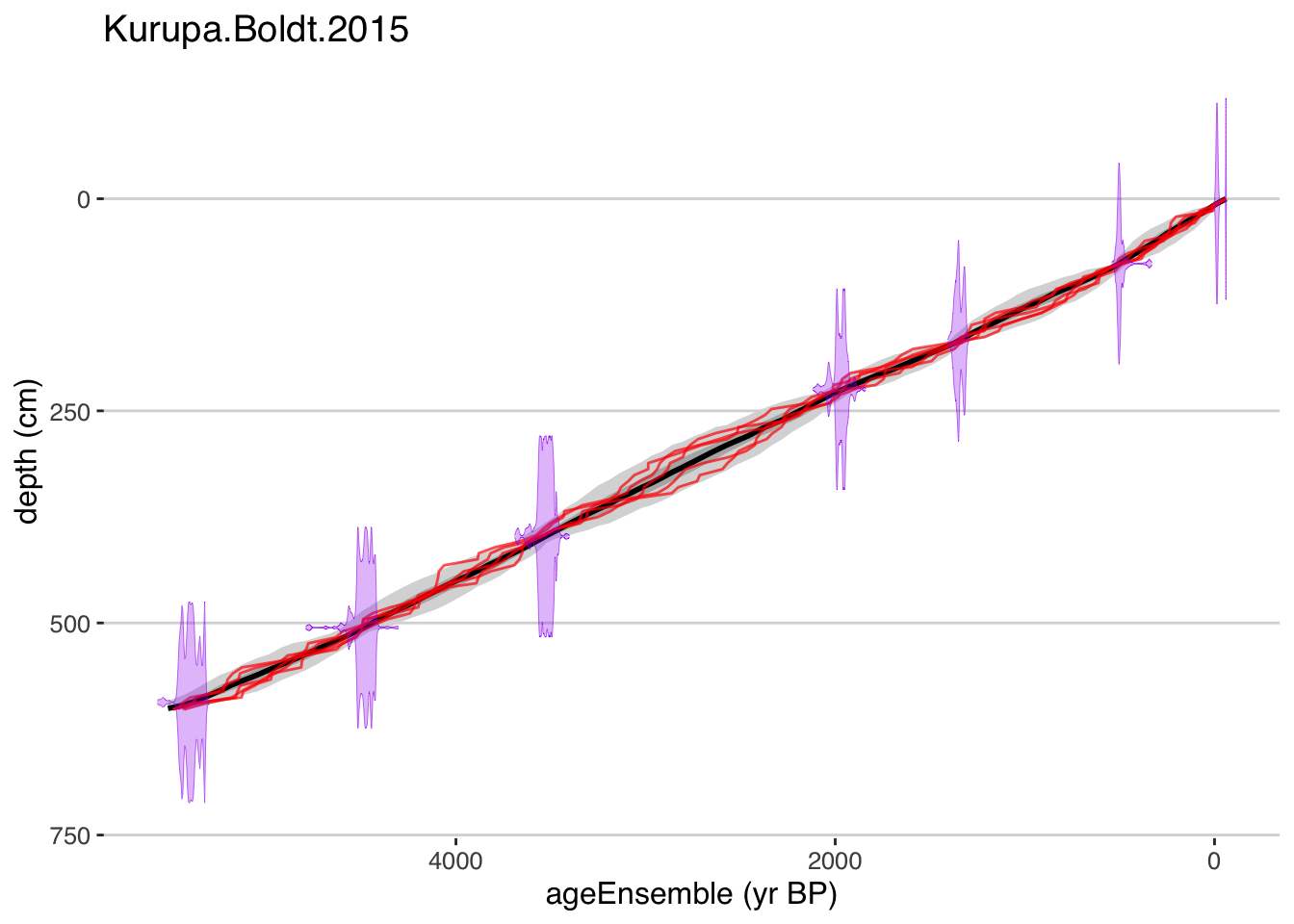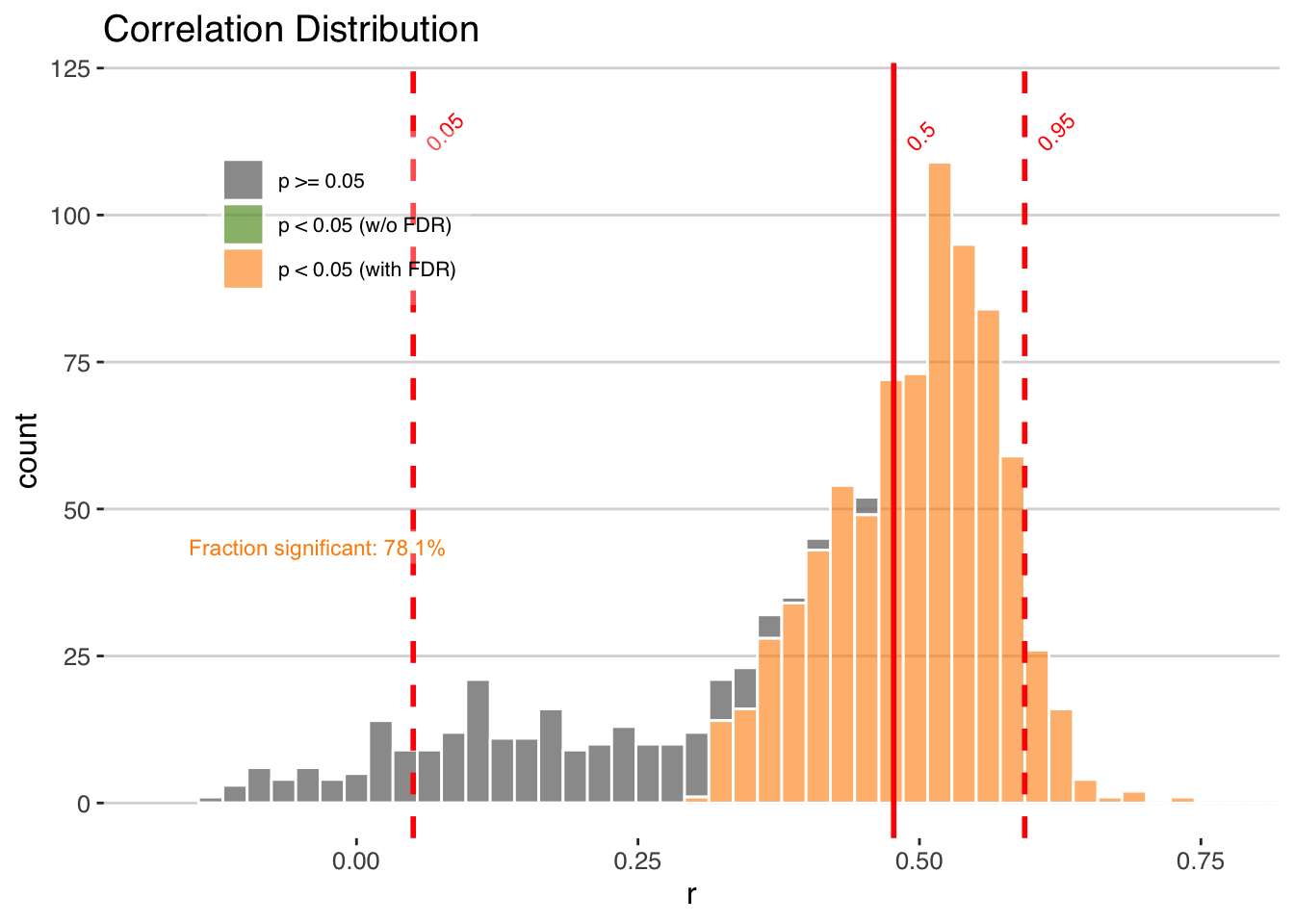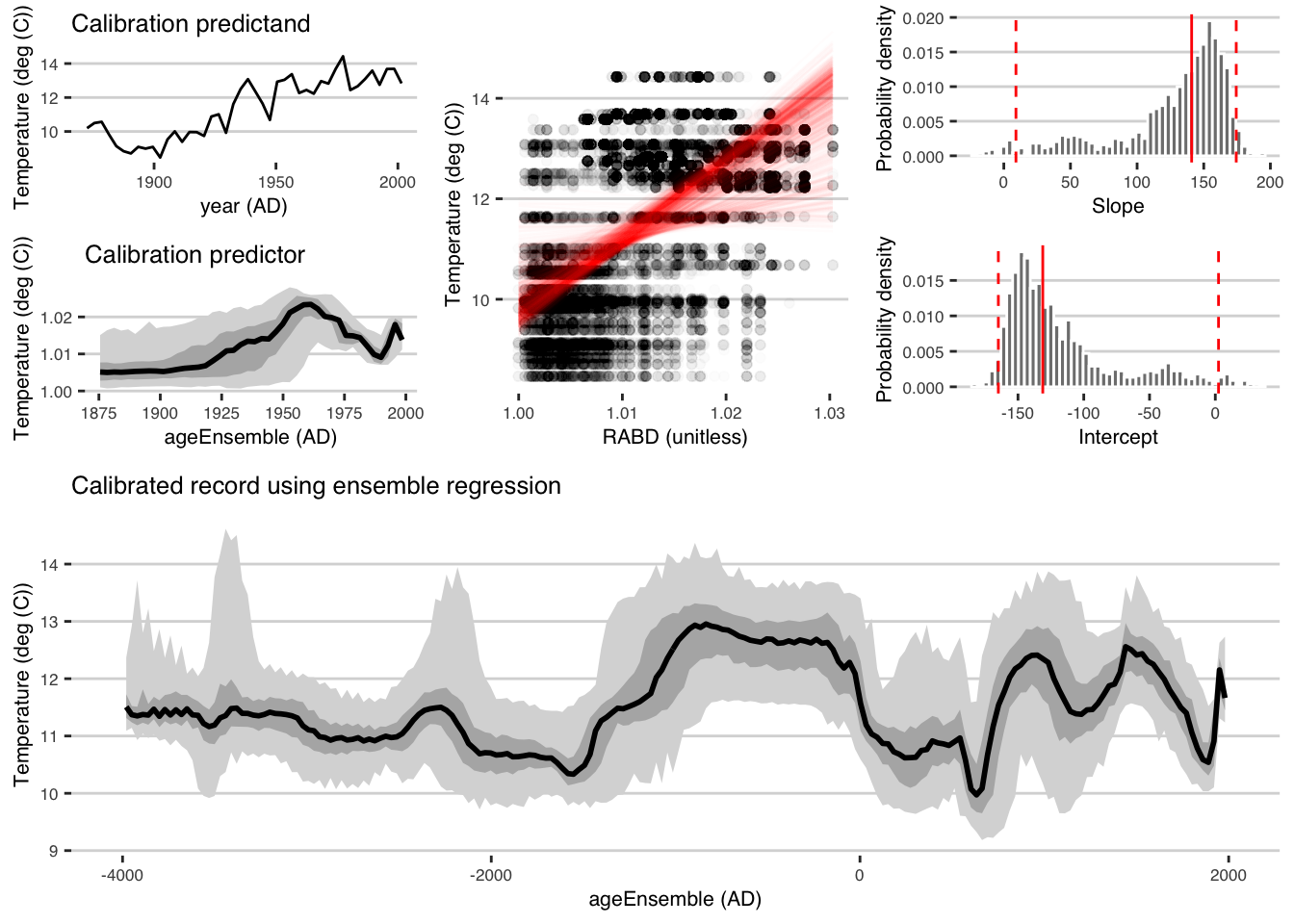# Chapter 7 Regression and Calibration-in-time

In this chapter, we will replicate the analysis of , performing age-uncertain calibration-in-time on a chlorophyll reflectance record from northern Alaska, using geoChronR.

The challenge of age-uncertain calibration-in-time is that age uncertainty affects both the calibration model (the relation between the proxy data and instrumental data) and the reconstruction (the timing of events in the reconstruction). geoChronR simplifies handling these issues.

library(lipdR) #to read and write LiPD files
library(geoChronR) #of course
library(ggplot2) #for plotting

## 7.1 Load the LiPD file

OK, we’ll begin by loading in the Kurupa Lake record from .

K <- lipdR::readLipd("http://lipdverse.org/geoChronR-examples/Kurupa.Boldt.2015.lpd")
##  "reading: Kurupa.Boldt.2015.lpd"

### 7.1.1 Check out the contents

sp <- plotSummary(K,paleo.data.var = "RABD",summary.font.size = 6)
##  "Found it! Moving on..."
##  "Found it! Moving on..."
##  "Found it! Moving on..."
##  "Found it! Moving on..."
##  "Found it! Moving on..."print(sp)
## TableGrob (4 x 4) "arrange": 4 grobs
##   z     cells    name                    grob
## 1 1 (1-1,1-2) arrange gTree[GRID.gTree.19188]
## 2 2 (1-2,3-4) arrange          gtable[layout]
## 3 3 (2-4,1-2) arrange          gtable[layout]
## 4 4 (3-4,3-4) arrange          gtable[layout]

## 7.2 Create an age model

K <- runBacon(K,
lab.id.var = 'labID',
age.14c.var = 'age14C',
age.14c.uncertainty.var = 'age14CUncertainty',
age.var = 'age',
age.uncertainty.var = 'ageUncertainty',
depth.var = 'depth',
reservoir.age.14c.var = NULL,
reservoir.age.14c.uncertainty.var = NULL,
rejected.ages.var = NULL,
bacon.acc.mean = 10,
bacon.thick = 7,
bacon.dir = "~/Cores",
suggest = FALSE,
close.connection = FALSE)

Exercise 7.1 In this chapter, the exercises will have conduct the analysis in a parallel universe, where you make different, but reasonable choices and see what happens.

First, create the best age model you can using either Bchron or Oxcal.

### 7.2.1 And plot the ensemble output

plotChron(K,age.var = "ageEnsemble",dist.scale = 0.2)
##  "Found it! Moving on..."
##  "Found it! Moving on..."
##  "plotting your chron ensemble. This make take a few seconds..."
## Scale for 'x' is already present. Adding another scale for 'x', which will replace the existing scale.Exercise 7.2 Plot your model. How does it compare to the Bacon model?

## 7.3 Prepare the data

### 7.3.1 Map the age ensemble to the paleodata table

This is to get ensemble age estimates for each depth in the paleoData measurement table

K <- mapAgeEnsembleToPaleoData(K,age.var = "ageEnsemble")
##  "Kurupa.Boldt.2015"
##  "Looking for age ensemble...."
##  "Found it! Moving on..."
##  "Found it! Moving on..."
##  "getting depth from the paleodata table..."
##  "Found it! Moving on..."

### 7.3.2 Select the paleodata age ensemble, and RABD data that we’d like to regress and calibrate

kae <-  selectData(K,"ageEnsemble")
##  "Found it! Moving on..."
rabd <- selectData(K,"RABD")
##  "Found it! Moving on..."

### 7.3.3 Now load in the instrumental data

kurupa.instrumental <- readr::read_csv("http://lipdverse.org/geoChronR-examples/KurupaInstrumental.csv")
## Rows: 134 Columns: 2
## ── Column specification ──────────────────────────────────────────────────────────────────────────────────────────────────────────
## Delimiter: ","
## dbl (2): Year (AD), JJAS Temperature (deg C)
##
## ℹ Use spec() to retrieve the full column specification for this data.
## ℹ Specify the column types or set show_col_types = FALSE to quiet this message.

kae$units ##  "yr BP" yep, we need to convert the units from BP to AD kae <- convertBP2AD(kae) ### 7.3.5 Create a “variable list” for the instrumental data kyear <- list() kyear$values <- kurupa.instrumental[,1]
kyear$variableName <- "year" kyear$units <- "AD"

kinst <- list()
kinst$values <- kurupa.instrumental[,2] kinst$variableName <- "Temperature"
kinst\$units <- "deg (C)"

Exercise 7.3 Repeat all of this prep work, including mapping the age uncertainties and extracting the variables.

### 7.3.6 Calculate an ensemble correlation between the RABD and local summer temperature data

corout <- corEns(kae,rabd,kyear,kinst,bin.step=2,percentiles = c(.05,.5,.95 ))

Exercise 7.4 Calculate the correlation ensemble, but you decide to use 5-year bins instead of 2-year bins.

### 7.3.7 And plot the output

Note that here we use the “Effective-N” significance option as we mimic the Boldt et al. (2015) paper.

plotCorEns(corout,significance.option = "eff-n")Mixed results. But encouraging enough to move forward.

Exercise 7.5 After reading Chapter ??, you know that the isospectral method is usually more reliable. Use that instead.

## 7.4 Perform ensemble regression

OK, you’ve convinced yourself that you want to use RABD to model temperature back through time. We can do this simply (perhaps naively) with regression, and lets do it with age uncertainty, both in the building of the model, and the reconstructing

regout <- regressEns(time.x = kae,
values.x = rabd,
time.y =kyear,
values.y =kinst,
bin.step=3,
gaussianize = FALSE,
recon.bin.vec = seq(-4010,2010,by=20))

Exercise 7.6 Again, use 5-year time steps, and also gaussianize to avoid the potential impact of skewed data

### 7.4.1 And plot the output

regPlots <- plotRegressEns(regout,alp = 0.01,font.size = 8)This result is consistent with that produced by Boldt et al., (2015), and was much simpler to produce with geoChronR.

## 7.5 Chapter project

Exercise 7.7 Plot your results. How did making different, reasonable (perhaps even better?) choices affect the final outcome of the regression?

### References

Boldt, Brandon R., Darrell S. Kaufman, Nicholas P. McKay, and Jason P. Briner. 2015. “Holocene Summer Temperature Reconstruction from Sedimentary Chlorophyll Content, with Treatment of Age Uncertainties, Kurupa Lake, Arctic Alaska.” The Holocene, January. https://doi.org/10.1177/0959683614565929.